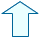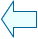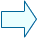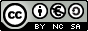Mass Metres, litres, kilograms

2 . Mass unit conversion.

The base unit of mass is the kilogram, it is the unit used more frequently.
We express the measurements of large masses in metric tonnes. When the mass is small, we express the measurement in grams and when it is very small we express it in milligrams.

Esta unidad interactiva requiere la máquina virtual de Java J2RE.

Volume unit conversion is done in the same way as the length and volume units and the units of our numeration system.
Each unit has a value ten times larger than the unit on the right and ten times smaller than the unit on the left.
You have to notice in which direction the unit jumps in order to move the decimal point in the same way.

 This activity shows a measurement expressed in a certain unit and on the right you will find the unit to do the conversion. Type the resulting number in the small window below. After typing the number hit Enter. If your answer is correct, this number will appear. If it isn't, try again.

When you click on Init, a different activity will appear, do several conversions until you master unit conversion.

Esta unidad interactiva requiere la máquina virtual de Java J2RE.

A tonne equals 1000 kilograms, so when converting tonnes into kilograms, we multiply it by 1000. In order to do this, you have to move the decimal point three steps to the right. When converting kilograms into tonnes, we divide it by 1000. In order to do this, you have to move the comma three steps to the left.Eduardo Barbero CorralSpanish Ministry of Education. Year 2007